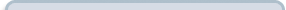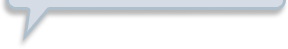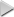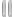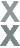# Simply Surveying

###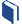Resources for this lesson: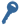Key Terms:

Statistical study
Population
Sample
Population parameter
Hypothesis

You will use your Algebra II Journalon this page.

> Glossary> Calculator Resources> Teacher Resources: Instructional NotesApply the Model

Allyson and Marissa have completed a statistical study about the average snowfall in the United States. The population they are studying is the states in the United States. A population in a statistical study is the complete set of people or things being studied. The sample they are looking at is the ten states they chose at random to compare to Maryland. A statistical sample is part of the population selected to represent the entire population.

The mean snowfall amount the girls calculated is a sample mean. The population mean and population standard deviation, which are also called a population parameter, were not used in their study. The mean average snowfall amount for the states in the United States is 28.15 inches, with a standard deviation of 27.437 inches.

##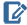Algebra II Journal: Reflection 1

Respond to the following reflection questions in your Algebra II Journaland submit to your teacher.

• How does the sample mean for the ten states (39.29 inches) compare to the population mean of 28.15 inches?
• How does the population mean of 28.15 inches compare to the average snowfall of Maryland (20.6 inches)?
• The population standard deviation is 27.437 inches. The sample standard deviation is 30.688 inches. What do these values say about the data?
• Allyson’s hypothesis was that Maryland gets more snow than any other state. Marissa’s hypothesis was that Maryland gets about the same amount of snow as the United States. (A hypothesis is a statement made about the population parameter.) Can either hypothesis be supported by the sample statistics? Use what you know about statistics to justify your answer.